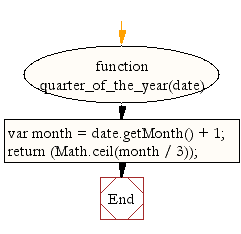# JavaScript: Get the quarter of the year

## JavaScript Datetime: Exercise-15 with Solution

Write a JavaScript function to get the quarter (1 to 4) of the year.

Test Data:
console.log(quarter_of_the_year(new Date(2015, 1, 21)));
2
console.log(quarter_of_the_year(new Date(2015, 10, 18)));
4

Sample Solution:-

HTML Code:

``````<!DOCTYPE html>
<html>
<head>
<meta charset="utf-8">
<title>JavaScript function to get the the quarter (1 to 4) of the year</title>
</head>
<body>

</body>
</html>
```
```

JavaScript Code:

``````function quarter_of_the_year(date)
{
var month = date.getMonth() + 1;
return (Math.ceil(month / 3));
}

console.log(quarter_of_the_year(new Date()));
console.log(quarter_of_the_year(new Date(2015, 1, 21)));
console.log(quarter_of_the_year(new Date(2015, 10, 18)));
```
```

Sample Output:

```2
1
4
```

Flowchart:Live Demo:

See the Pen JavaScript - Get the quarter of the year-date-ex- 15 by w3resource (@w3resource) on CodePen.

Improve this sample solution and post your code through Disqus

What is the difficulty level of this exercise?

﻿

## JavaScript: Tips of the Day

Convert tabs to spaces, where each tab corresponds to count spaces

Example:

```const tips_expandTabs = (str, count) => str.replace(/\t/g, ' '.repeat(count));
console.log(tips_expandTabs('\t\tw3resource', 4));
```

Output:

```"        w3resource"
```